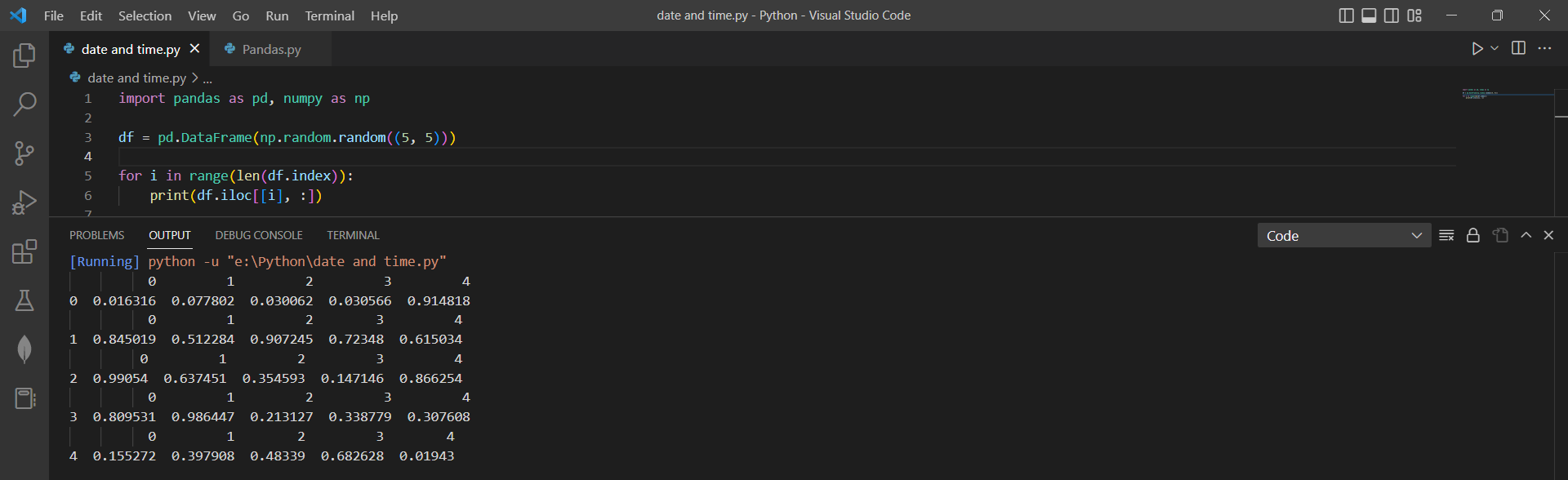# Iteration over rows using "iloc" function in pandasby vigneshchennai74Updated: Feb 23, 2023Solution Kit

The iloc function in Pandas is often used to select specific rows or columns from a DataFrame. It works by specifying the elements' integer-based row and column indices to select.

To iterate over rows in a DataFrame using iloc, you can use a for loop that iterates over the range of row indices. iloc function helps in many ways:

• Selecting rows or columns by index number: iloc allows you to retrieve rows or columns from a DataFrame based on their position in the DataFrame, rather than their labels. For example, you can use df.iloc to select the first row of a DataFrame, or df.iloc[:, 0] to select the first column.
• Slicing rows or columns: iloc also allows you to slice rows or columns of a DataFrame by their index. For example, you can use df.iloc[1:3] to select rows 1 and 2, or df.iloc[:, 1:3] to select columns 1 and 2.

Pandas rely heavily on NumPy for their underlying data storage and many of its numerical operations. Pandas DataFrames are built on top of NumPy arrays and can be converted to NumPy arrays using the to_numpy() method. Similarly, many Pandas functions accept NumPy arrays as input or return NumPy arrays as output. NumPy and Pandas are powerful and widely-used libraries in the Python data analysis ecosystem. While they have different primary focuses, they can be used together to perform various data analysis tasks.

range(len) is a built-in Python function that returns an iterator over a sequence of numbers from 0 to (but not including) the given length. The len function is used to get the sequence length or container being iterated over. range(len) in this way can be useful when you need to iterate over the indices of a list or other sequence rather than the elements themselves.

An iloc function is a powerful tool for selecting and manipulating subsets of data in a Pandas DataFrame based on their position in the DataFrame.Preview of the output that you will get on running this code.

### Code

In this code we have used Iloc() function in Pandas Library

1. Copy this code using "Copy" button above and paste it in your Python ide
2. Import Pandas library of python.
3. Run the code to collect a Multiple columns

I hope you have found this useful. I have added the dependent library and version information in the following section.

I found this code snippet by searching "Iterate over rows of pandas dataframe " in kandi. you can try any use case.

### Environment Tested

In this solution we have used the following versions. Be mindful to change when working with other versions.

1. This solution is tested using Vscode version 1.75.1
2. This solution is created and tested using Python version 3.7.15
3. This solution is Tested using Pandas version 1.3.5
4. This solution is Tested using numpy version 1.21.6

Using this solution we can able to Iterate the rows in a Dataframe using Pandas library in python with simple Steps. This process also facilities an easy to use, hassle free method to create a hands-on working version of code which would help us to Iterate the rows in a Dataframe in Python.

### Dependent Library

pandasby pandas-dev

Python38689Version:v2.0.2Flexible and powerful data analysis / manipulation library for Python, providing labeled data structures similar to R data.frame objects, statistical functions, and much more

Support
Quality
Security
Reuse

pandasby pandas-dev

Python38689Version:v2.0.2License: Permissive (BSD-3-Clause)

Flexible and powerful data analysis / manipulation library for Python, providing labeled data structures similar to R data.frame objects, statistical functions, and much more
Support
Quality
Security
Reuse

numpyby numpy

Python23755Version:v1.25.0rc1The fundamental package for scientific computing with Python.

Support
Quality
Security
Reuse

numpyby numpy

Python23755Version:v1.25.0rc1License: Permissive (BSD-3-Clause)

The fundamental package for scientific computing with Python.
Support
Quality
Security